# Statements

## BY

### Overview of the BY Statement

Orders the output according to the BY groups.
BY <DESCENDING> variable-1
<… <DESCENDING> variable-n>
<NOTSORTED>;

### Required Arguments

variable
specifies the variable that the procedure uses to form BY groups. You can specify more than one variable. If you do not use the NOTSORTED option in the BY statement, then either the observations in the data set must be sorted by all the variables that you specify, or they must be indexed appropriately. Variables in a BY statement are called BY variables.

### Optional Arguments

DESCENDING
specifies that the observations are sorted in descending order by the variable that immediately follows the word DESCENDING in the BY statement.
NOTSORTED
specifies that observations are not necessarily sorted in alphabetic or numeric order. The observations are grouped in another way (for example, chronological order).
The requirement for ordering or indexing observations according to the values of BY variables is suspended for BY-group processing when you use the NOTSORTED option. In fact, the procedure does not use an index if you specify NOTSORTED. The procedure defines a BY group as a set of contiguous observations that have the same values for all BY variables. If observations with the same values for the BY variables are not contiguous, then the procedure treats each contiguous set as a separate BY group.
You cannot use the NOTSORTED option in a PROC SORT step.
Note: You cannot use the GROUPFORMAT option, which is available in the BY statement in a DATA step, in a BY statement in any PROC step.

### BY-Group Processing

Procedures create output for each BY group. For example, the elementary statistics procedures and the scoring procedures perform separate analyses for each BY group. The reporting procedures produce a report for each BY group.
Note: All Base SAS procedures except PROC PRINT process BY groups independently. PROC PRINT can report the number of observations in each BY group as well as the number of observations in all BY groups. Similarly, PROC PRINT can sum numeric variables in each BY group and across all BY groups.
You can use only one BY statement in each PROC step. When you use a BY statement, the procedure expects an input data set that is sorted by the order of the BY variables or one that has an appropriate index. If your input data set does not meet these criteria, then an error occurs. Either sort it with the SORT procedure or create an appropriate index on the BY variables.
Depending on the order of your data, you might need to use the NOTSORTED or DESCENDING option in the BY statement in the PROC step.

### Formatting BY-Variable Values

When a procedure is submitted with a BY statement, the following actions are taken with respect to processing of BY groups:
1. The procedure determines whether the data is sorted by the internal (unformatted) values of the BY variable(s).
2. The procedure determines whether a format has been applied to the BY variable(s). If the BY variable is numeric and has no user-applied format, then the BEST12. format is applied for the purpose of BY-group processing.
3. The procedure continues adding observations to the current BY group until both the internal and the formatted values of the BY variable or variables change.
This process can have unexpected results if, for example, nonconsecutive internal BY values share the same formatted value. In this case, the formatted value is represented in different BY groups. Alternatively, if different consecutive internal BY values share the same formatted value, then these observations are grouped into the same BY group.

### BY Variables That Have Different Lengths in Two Data Sets

When a procedure has a BY statement and two input data sets, one of the input data sets is called the primary data set and the other is called the secondary data set. The primary data set is usually, but not always, the DATA= data set. A BY statement always applies to the primary data set. The variables in the BY statement must appear in the primary data set.
Each procedure determines whether a BY statement applies to a secondary data set, and performs one of the following actions:
• The procedure might always apply the BY statement to the secondary data set. In this case, one or more variables in the BY statement must appear in the secondary data set.
• The procedure might never apply the BY statement to the secondary data set. In this case the variables in the BY statement are not required in the secondary data set.
• The procedure might check whether the BY variables are in the secondary data set. If none of the BY variables are in the secondary data set, then BY processing does not occur for the secondary data set. If one or more of the BY variables are in the secondary data set, and they match the BY variables in the primary data set, then BY processing is done for the secondary data set. If some but not all BY variables are in the secondary data set, then the procedure might issue an error message and quit. Or, it might take some other action described in the documentation for that particular procedure.
If the BY statement is applied to the secondary data set, then each BY variable that exists on both the data sets must have the same type, character or numeric, in both data sets. The BY variables are required to have either the same formatted value or the same unformatted value. Formatted values match only if both the formatted lengths and the formatted values are the same. Unformatted values are not required to have the same length in order to match. The unformatted character values match if the unformatted values are the same after stripping the trailing blanks. The unformatted doubles match if they have the same value.
A secondary data set does not need to have all of the BY variables that are in the primary data set. A procedure can define a subset of the BY variables for the secondary data set. For example, if the primary data set has the BY variables A,B,C,D, then the procedure can define the following BY variables on the secondary data set:
• A
• A,B
• A,B,C
• A,B,C,D
If both the primary and secondary data sets have the same number of BY variables, and all the BY variables have the same byte lengths and format lengths, then either the unformatted values or the formatted values in the BY buffer (for all of the BY variables) have to match. If they do not match, then each variable is compared. The formatted values of each variable are compared first. The formatted lengths have to match, and the formatted values have to match. If the formatted lengths and values do not match, then the unformatted values are compared even if the byte lengths are different.
If corresponding character variable lengths differ, then the longer character variable can contain only trailing blanks for the extra characters. If the lengths of the character variables are different, then the values match as long as they are the same after stripping the trailing blanks. For example, ‘ABCD’ in the primary data set matches ‘ABCD ’ in the secondary data set. If the secondary data set contained ‘ABCDEF’, then they would not match.

### Base SAS Procedures That Support the BY Statement

 CALENDAR REPORT (nonwindowing environment only) CHART SORT (required) COMPARE STANDARD CORR SUMMARY FREQ TABULATE MEANS TIMEPLOT PLOT TRANSPOSE PRINT UNIVARIATE RANK
Note: In the SORT procedure, the BY statement specifies how to sort the data. In the other procedures, the BY statement specifies how the data is currently sorted.

### Example

This example uses a BY statement in a PROC PRINT step. There is output for each value of the BY variable Year. The DEBATE data set is created in
```options nodate pageno=1 linesize=64
pagesize=40;
proc print data=debate noobs;
by year;
title 'Printing of Team Members';
title2 'by Year';
run;```## FREQ

### Overview of the FREQ Statement

Treats observations as if they appear multiple times in the input data set.
You can use a WEIGHT statement and a FREQ statement in the same step of any procedure that supports both statements.
FREQ variable;

### Required Arguments

variable
specifies a numeric variable whose value represents the frequency of the observation. If you use the FREQ statement, then the procedure assumes that each observation represents n observations, where n is the value of variable. If variable is not an integer, then SAS truncates it. If variable is less than 1 or is missing, then the procedure does not use that observation to calculate statistics. If a FREQ statement does not appear, then each observation has a default frequency of 1.
The sum of the frequency variable represents the total number of observations.

### Procedures That Support the FREQ Statement

• CORR
• MEANS or SUMMARY
• REPORT
• STANDARD
• TABULATE
• UNIVARIATE

### Example

The data in this example represents a ship's course and speed (in nautical miles per hour), recorded every hour. The frequency variable Hours represents the number of hours that the ship maintained the same course and speed. Each of the following PROC MEANS steps calculates average course and speed. The different results demonstrate the effect of using Hours as a frequency variable.
The following PROC MEANS step does not use a frequency variable:
```options nodate pageno=1 linesize=64 pagesize=40;

data track;
input Course Speed Hours @@;
datalines;
30  4  8 50 7 20
75 10 30 30 8 10
80  9 22 20 8 25
83 11  6 20 6 20
;```
```proc means data=track maxdec=2 n mean;
var course speed;
title 'Average Course and Speed';
run;```
Without a frequency variable, each observation has a frequency of 1, and the total number of observations is 8.The second PROC MEANS step uses Hours as a frequency variable:
```   proc means data=track maxdec=2 n mean;
var course speed;
freq hours;
title 'Average Course and Speed';
run;```
When you use Hours as a frequency variable, the frequency of each observation is the value of Hours. The total number of observations is 141 (the sum of the values of the frequency variable).## QUIT

### Overview of the QUIT Statement

Executes any statements that have not executed and ends the procedure.
QUIT;

• CATALOG
• DATASETS
• PLOT
• SQL

## WEIGHT

### Overview of the WEIGHT Statement

Specifies weights for analysis variables in the statistical calculations.
You can use a WEIGHT statement and a FREQ statement in the same step of any procedure that supports both statements.
WEIGHT variable;

### Required Arguments

variable
specifies a numeric variable whose values weight the values of the analysis variables. The values of the variable do not have to be integers. The behavior of the procedure when it encounters a nonpositive weight variable value is as follows:
Different behavior for nonpositive values is discussed in the WEIGHT statement syntax under the individual procedure.
Before Version 7 of SAS, no Base SAS procedure excluded the observations with missing weights from the analysis. Most SAS/STAT procedures, such as PROC GLM, have always excluded not only missing weights but also negative and zero weights from the analysis. You can achieve this same behavior in a Base SAS procedure that supports the WEIGHT statement by using the EXCLNPWGT option in the PROC statement.
 Weight value Procedure 0 Counts the observation in the total number of observations less than 0 Converts the weight value to zero and counts the observation in the total number of observations missing Excludes the observation from the analysis
The procedure substitutes the value of the WEIGHT variable for, which appears in

### Procedures That Support the WEIGHT Statement

• CORR
• FREQ
• MEANS or SUMMARY
• REPORT
• STANDARD
• TABULATE
• UNIVARIATE
Note: In PROC FREQ, the value of the variable in the WEIGHT statement represents the frequency of occurrence for each observation. For more information, see the information about PROC FREQ in the Base SAS(R) 9.3 Procedures Guide: Statistical Procedures .

### Calculating Weighted Statistics

The procedures that support the WEIGHT statement also support the VARDEF= option, which lets you specify a divisor to use in the calculation of the variance and standard deviation.
By using a WEIGHT statement to compute moments, you assume that the ith observation has a variance that is equal to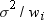. When you specify VARDEF=DF (the default), the computed variance is a weighted least squares estimate of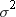. Similarly, the computed standard deviation is an estimate of σ. Note that the computed variance is not an estimate of the variance of the ith observation, because this variance involves the observation's weight, which varies from observation to observation.
If the values of your variable are counts that represent the number of occurrences of each observation, then use this variable in the FREQ statement rather than in the WEIGHT statement. In this case, because the values are counts, they should be integers. (The FREQ statement truncates any noninteger values.) The variance that is computed with a FREQ variable is an estimate of the common varianceof the observations.
Note: If your data comes from a stratified sample where the weights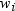represent the strata weights, then neither the WEIGHT statement nor the FREQ statement provides appropriate stratified estimates of the mean, variance, or variance of the mean. To perform the appropriate analysis, consider using PROC SURVEYMEANS, which is a SAS/STAT procedure that is documented in the Base SAS(R) 9.3 Procedures Guide: Statistical Procedures .

### Weighted Statistics Example

As an example of the WEIGHT statement, suppose 20 people are asked to estimate the size of an object 30 cm wide. Each person is placed at a different distance from the object. As the distance from the object increases, the estimates should become less precise.
The SAS data set SIZE contains the estimate (ObjectSize) in centimeters at each distance (Distance) in meters and the precision (Precision) for each estimate. Notice that the largest deviation (an overestimate by 20 cm) came at the greatest distance (7.5 meters from the object). As a measure of precision, 1/Distance, gives more weight to estimates that were made closer to the object and less weight to estimates that were made at greater distances.
The following statements create the data set SIZE:
```options nodate pageno=1 linesize=64 pagesize=60;

data size;
input Distance ObjectSize @@;
Precision=1/distance;
datalines;
1.5 30 1.5 20 1.5 30 1.5 25
3   43 3   33 3   25 3   30
4.5 25 4.5 36 4.5 48 4.5 33
6   43 6   36 6   23 6   48
7.5 30 7.5 25 7.5 50 7.5 38
;```
The following PROC MEANS step computes the average estimate of the object size while ignoring the weights. Without a WEIGHT variable, PROC MEANS uses the default weight of 1 for every observation. Thus, the estimates of object size at all distances are given equal weight. The average estimate of the object size exceeds the actual size by 3.55 cm.
```proc means data=size maxdec=3 n mean var stddev;
var objectsize;
title1 'Unweighted Analysis of the SIZE Data Set';
run;```The next two PROC MEANS steps use the precision measure (Precision) in the WEIGHT statement and show the effect of using different values of the VARDEF= option. The first PROC step creates an output data set that contains the variance and standard deviation. If you reduce the weighting of the estimates that are made at greater distances, the weighted average estimate of the object size is closer to the actual size.
```proc means data=size maxdec=3 n mean var stddev;
weight precision;
var objectsize;
output out=wtstats var=Est_SigmaSq std=Est_Sigma;
title1 'Weighted Analysis Using Default VARDEF=DF';
run;

proc means data=size maxdec=3 n mean var std
vardef=weight;
weight precision;
var objectsize;
title1 'Weighted Analysis Using VARDEF=WEIGHT';
run;```
The variance of the ith observation is assumed to beand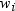is the weight for the ith observation. In the first PROC MEANS step, the computed variance is an estimate of. In the second PROC MEANS step, the computed variance is an estimate of, whereis the average weight. For large n, this value is an approximate estimate of the variance of an observation with average weight.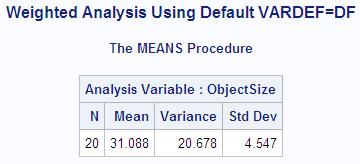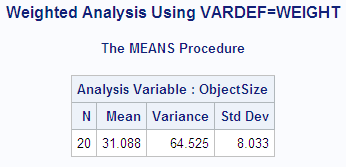The following statements create and print a data set with the weighted variance and weighted standard deviation of each observation. The DATA step combines the output data set that contains the variance and the standard deviation from the weighted analysis with the original data set. The variance of each observation is computed by dividing Est_SigmaSq (the estimate offrom the weighted analysis when VARDEF=DF) by each observation's weight (Precision). The standard deviation of each observation is computed by dividing Est_Sigma (the estimate offrom the weighted analysis when VARDEF=DF) by the square root of each observation's weight (Precision).
```data wtsize(drop=_freq_ _type_);
set size;
if _n_=1 then set wtstats;
Est_VarObs=est_sigmasq/precision;
Est_StdObs=est_sigma/sqrt(precision);

proc print data=wtsize noobs;
title 'Weighted Statistics';
by distance;
format est_varobs est_stdobs
est_sigmasq est_sigma precision 6.3;
run;```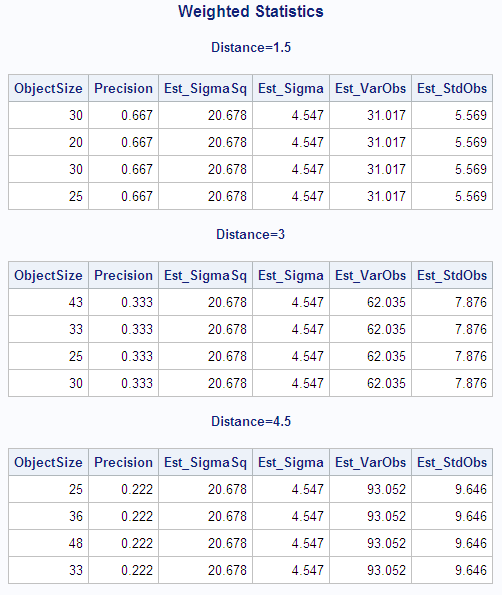## WHERE

### Overview of the WHERE Statement

Subsets the input data set by specifying certain conditions that each observation must meet before it is available for processing.
WHERE where-expression;

### Required Arguments

where-expression
is a valid arithmetic or logical expression that generally consists of a sequence of operands and operators. See SAS Statements: Reference for more information about where processing.

### Procedures That Support the WHERE Statement

You can use the WHERE statement with any of the following Base SAS procedures that read a SAS data set:
 CALENDAR RANK CHART REPORT COMPARE SORT CORR SQL DATASETS (APPEND statement) STANDARD FREQ TABULATE MEANS or SUMMARY TIMEPLOT PLOT TRANSPOSE PRINT UNIVARIATE

### Details

• The CALENDAR and COMPARE procedures and the APPEND statement in PROC DATASETS accept more than one input data set. See the documentation for the specific procedure for more information.
• To subset the output data set, use the WHERE= data set option:
```proc report data=debate nowd
out=onlyfr(where=(year='1'));
run;```
```   options nodate pageno=1 linesize=64
```   proc print data=debate noobs;